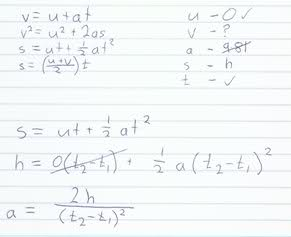# Kinemetics Question - theoretical subsitution

• Pardicer

## Homework Statement

This is for personal study and as I am on study leave its a bit hard to contact a teacher.I'm unsure as to why you would individually square the times before subtracting as the actual time is (t2-t1) substituted into time on the equation, squaring before will result in a larger value (small value for acceleration though). It is possible the teacher merely got it wrong (has been known to happen).

s=ut + (1/2)at^2

## The Attempt at a SolutionLast edited:

Your equation,  h = 0(t2-t1) + (1/2)a(t2-t1)2  is in error.

The velocity, u1, at time, t1 is not zero. This velocity is give by:
u1 = u0 + a(t1-t0) = 0 + a t1 = a t1 .​

∴ h = u1(t2-t1) + (1/2)a(t2-t1)2
= (a t1)(t2-t1) + (1/2)a(t2-t1)2

If you multiply this all out, combine like terms and then factor it, you will find that your teacher did indeed give the correct answer.

BTW: There is an easier way to come up with his answer. I thought it better to use what you started with.